# Matrix Question!eigenvectors and eigenvalues for symmetric matrix?

Suppose A is a symmetric N X N matrix with eigenvectors vi, i = 1; 2; 3 ...N with
corresponding eigenvalues ?i, i = 1; 2; 3 ...N.
Pick any two distinct eigenvalues (assuming such a pair exists). Let's call them ?1 and
?2 and their corresponding eigenvectors v1 and v2.
Write down the matrix equations that show that v1 and v2 are eigenvectors of A.

This Homework Help Question: "Matrix Question!eigenvectors and eigenvalues for symmetric matrix?" No answers yet.

We need 10 more requests to produce the answer to this homework help question. Share with your friends to get the answer faster!

0 /10 have requested the answer to this homework help question.

Once 10 people have made a request, the answer to this question will be available in 1-2 days.
All students who have requested the answer will be notified once they are available.
Similar Homework Help Questions
• ### Math problem ty~

Suppose A is a symmetric N N matrix with eigenvectors vi, i = 1; 2; 3 N withcorresponding eigenvalues i, i = 1; 2; 3 N.Pick any two distinct eigenvalues (assuming such a pair exists). Let's call them 1 and2 and their corresponding eigenvectors v1 and v2.(a) Write down the matrix equations that show that v1 and v2 are eigenvectors of A.(b) Compute the transpose of the equation satised by v2.(c) Multiply, from the right, the result of part (b)...

• ### 8. Find a symmetric 3 x 3 matrix with eigenvalues 11, 12 , and , 13...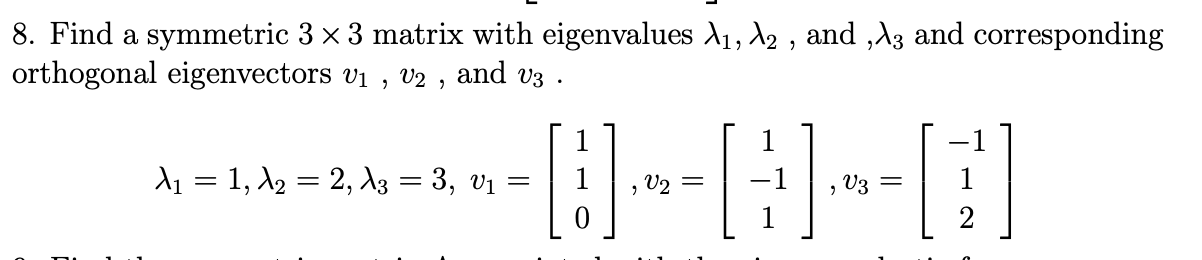8. Find a symmetric 3 x 3 matrix with eigenvalues 11, 12 , and , 13 and corresponding orthogonal eigenvectors vi , V2 , and V3 1 11 = 1, 12 = 2, 13 = 3, vi -=[:)--[:)--[;)] 1

• ### Question 2 (1 point) 8 -18 Find the eigenvalues and eigenvectors of the matrix A =...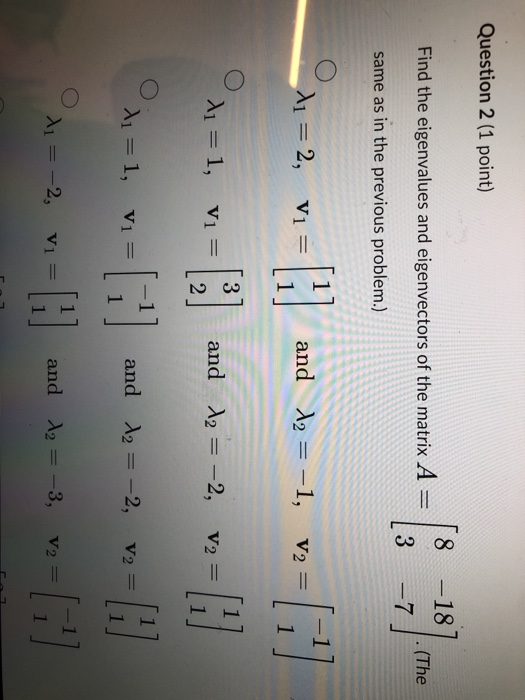Question 2 (1 point) 8 -18 Find the eigenvalues and eigenvectors of the matrix A = 18] (The 3 -7 same as in the previous problem.) di = 2, V1 =  and 12 = -1, V2 = -   3 21 = 1, V1 = ܒܗ ܟܬ and 12 = -2, V2 = 2 x = 1, V1 = and 12 = -2, V2 = [11 11 x = -2, Vi and 12 = -3, V2 

• ### Show that any two eigenvectors of the symmetric matrix corresponding to distinct eigenvalues are orthogonal. -1...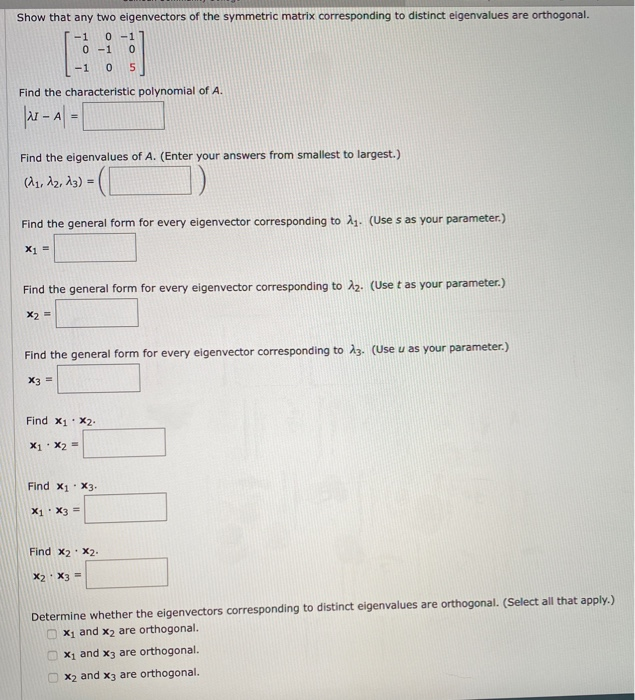Show that any two eigenvectors of the symmetric matrix corresponding to distinct eigenvalues are orthogonal. -1 0 -1 0-1 0 - 1 0 5 Find the characteristic polynomial of A. - A - Find the eigenvalues of A. (Enter your answers from smallest to largest.) (1, 12, 13) = ]) Find the general form for every eigenvector corresponding to N. (Uses as your parameter.) X1 = Find the general form for every eigenvector corresponding to 12. (Use t as your...

• ### [10 pointsjConsider an orthogonal matrix Q, which has two nonzero orthogonal eigenvectors v1 and ...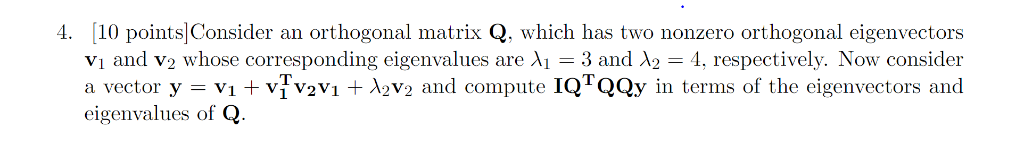[10 pointsjConsider an orthogonal matrix Q, which has two nonzero orthogonal eigenvectors v1 and v2 whose corresponding eigenvalues are λι = 3 and λ2-4, respectively. Now consider a vector y = Vi + vȚvayı + λ2V2 and compute 1QTQQy in terms of the eigenvectors and eigenvalues of Q 4. [10 pointsjConsider an orthogonal matrix Q, which has two nonzero orthogonal eigenvectors v1 and v2 whose corresponding eigenvalues are λι = 3 and λ2-4, respectively. Now consider a vector y =...

• ### (1 point) Suppose that the matrix A has the following eigenvalues and eigenvectors: 4 = 2...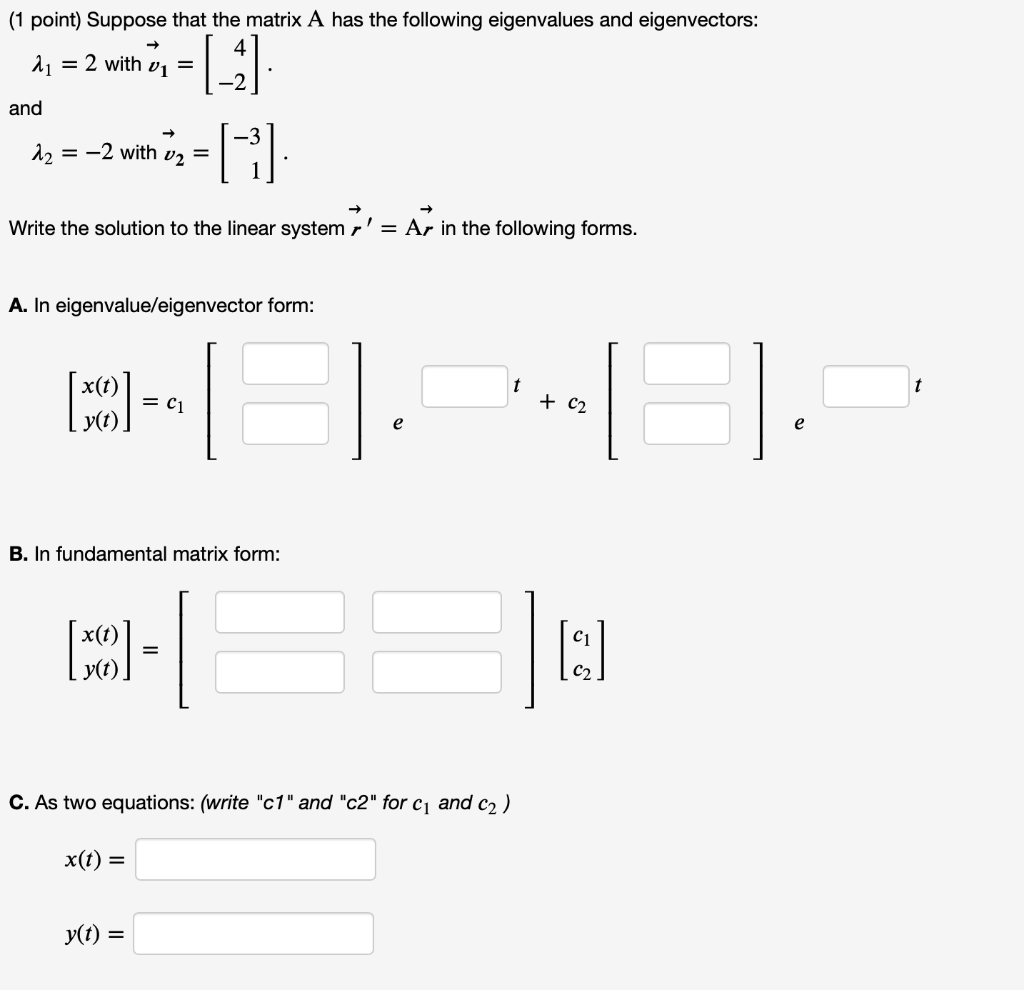(1 point) Suppose that the matrix A has the following eigenvalues and eigenvectors: 4 = 2 with vi = and |_ G 12 = -2 with v2 = Write the solution to the linear system r' = Ar in the following forms. A. In eigenvalue/eigenvector form: x(t) (50) = C1 + C2 e e B. In fundamental matrix form: (MCO) = I: C. As two equations: (write "c1" and "c2" for C1 and c2) x(t) = yt) =

• ### Suppose that the matrix A A has the following eigenvalues and eigenvectors: (1 point) Suppose that...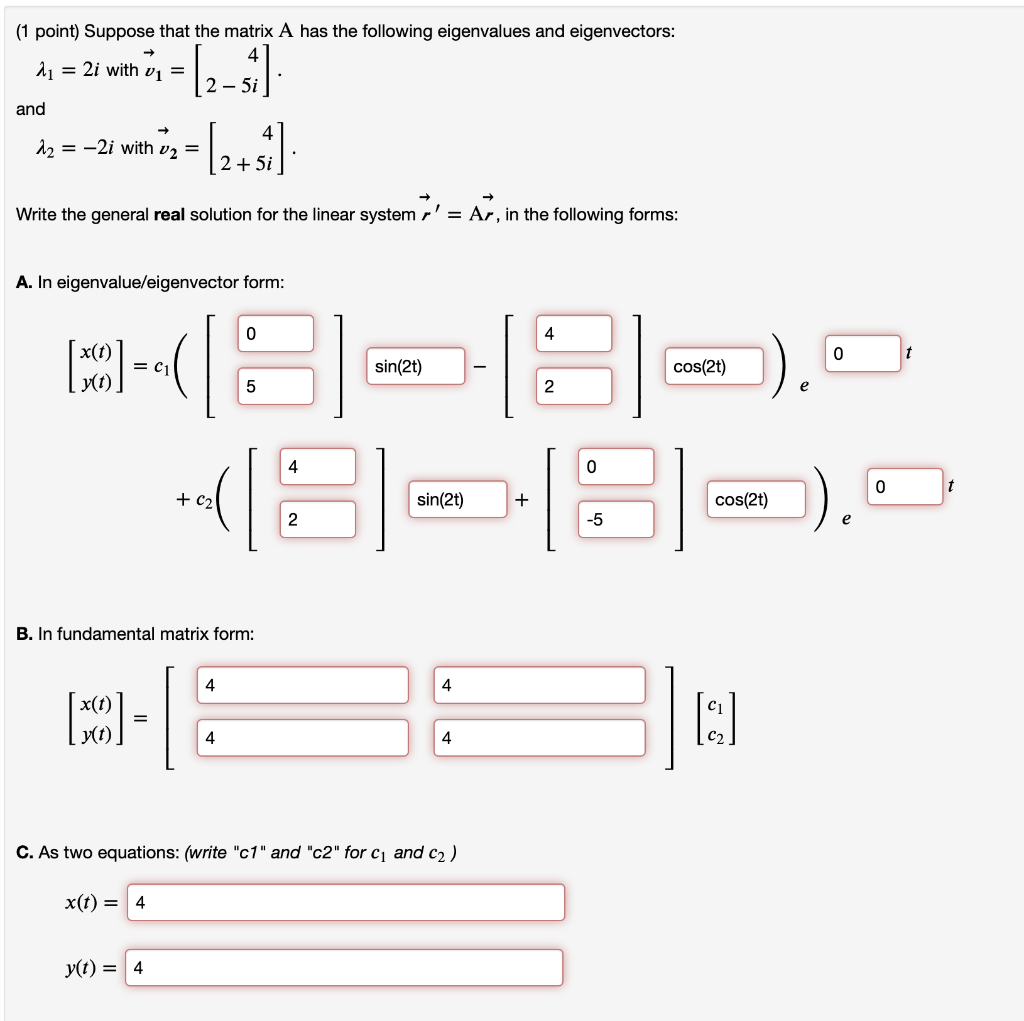Suppose that the matrix A A has the following eigenvalues and eigenvectors: (1 point) Suppose that the matrix A has the following eigenvalues and eigenvectors: 2 = 2i with v1 = 2 - 5i and - 12 = -2i with v2 = (2+1) 2 + 5i Write the general real solution for the linear system r' = Ar, in the following forms: A. In eigenvalue/eigenvector form: 0 4 0 t MODE = C1 sin(2t) cos(2) 5 2 4 0 0...

• ### linear algebra Show that any two eigenvectors of the symmetric matrix corresponding to distinct eigenvalues are...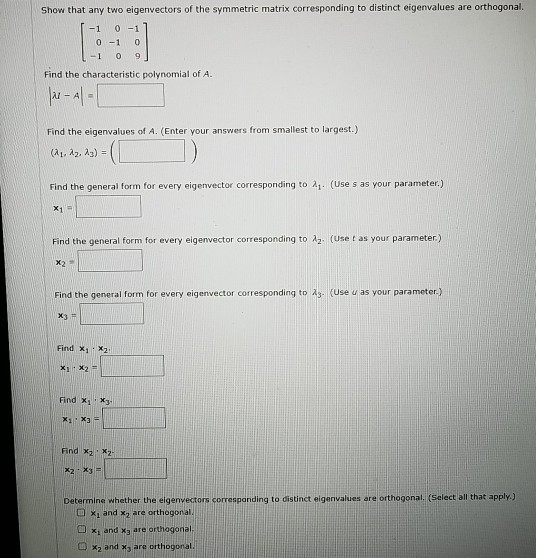linear algebra Show that any two eigenvectors of the symmetric matrix corresponding to distinct eigenvalues are orthogonal -1 0-1 0-1 0 - 1 0 9 1 Find the characteristic polynomial of A. |x - Al- Find the eigenvalues of A. (Enter your answers from smallest to largest.) (21, 22, 23) Find the general form for every eigenvector corresponding to 21. (Uses as your parameter.) X1 - Find the general form for every elge vector corresponding to Az. (Uset as your...

• ### Show that any two eigenvectors of the symmetric matrix corresponding to distinct eigenvalues are orthogonal. -1...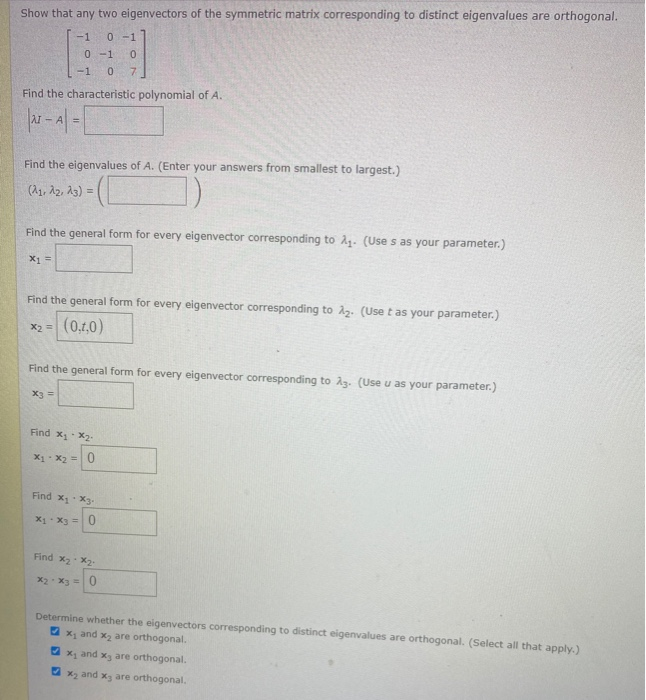Show that any two eigenvectors of the symmetric matrix corresponding to distinct eigenvalues are orthogonal. -1 0-1 0-1 0 -107 Find the characteristic polynomial of A. far - 41 - Find the eigenvalues of A. (Enter your answers from smallest to largest.) (11, 12, 13) = Find the general form for every eigenvector corresponding to 11. (Uses as your parameter.) X1 = Find the general form for every eigenvector corresponding to 12. (Use t as your parameter.) x2 = (0.t,0)...

• ### DETAILS LARLINALG8 7.3.033. Show that any two eigenvectors of the symmetric matrix A corresponding to distinct...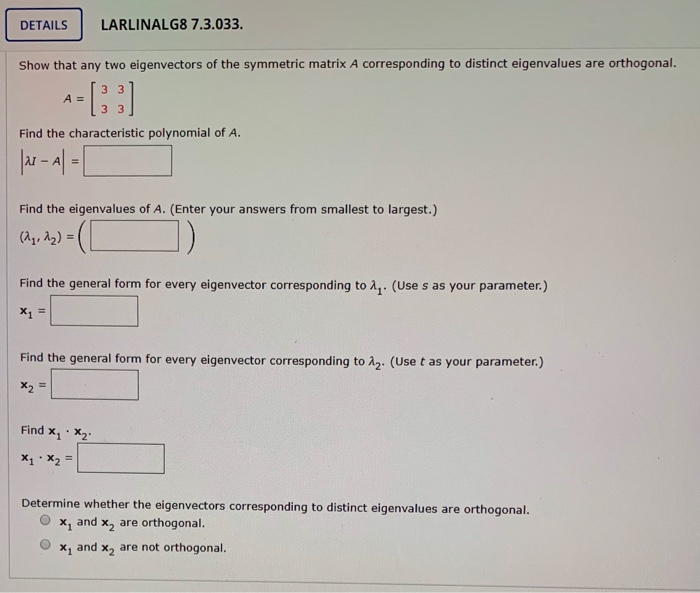DETAILS LARLINALG8 7.3.033. Show that any two eigenvectors of the symmetric matrix A corresponding to distinct eigenvalues are orthogonal. 3 A = Find the characteristic polynomial of A. |u-A=1 Find the eigenvalues of A. (Enter your answers from smallest to largest.) (14, 12) = Find the general form for every eigenvector corresponding to 1. (Use s as your parameter.) X1 = Find the general form for every eigenvector corresponding to 12. (Use t as your parameter.) X2 = Find x,...

Free Homework App